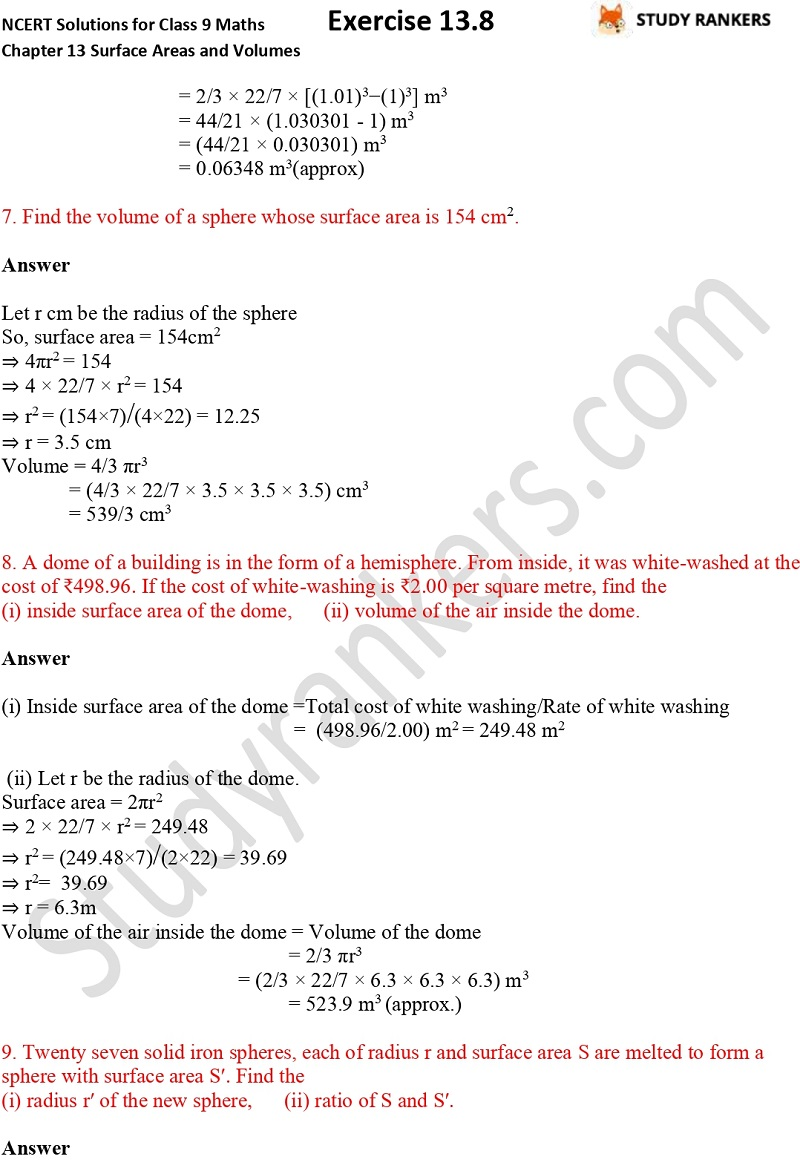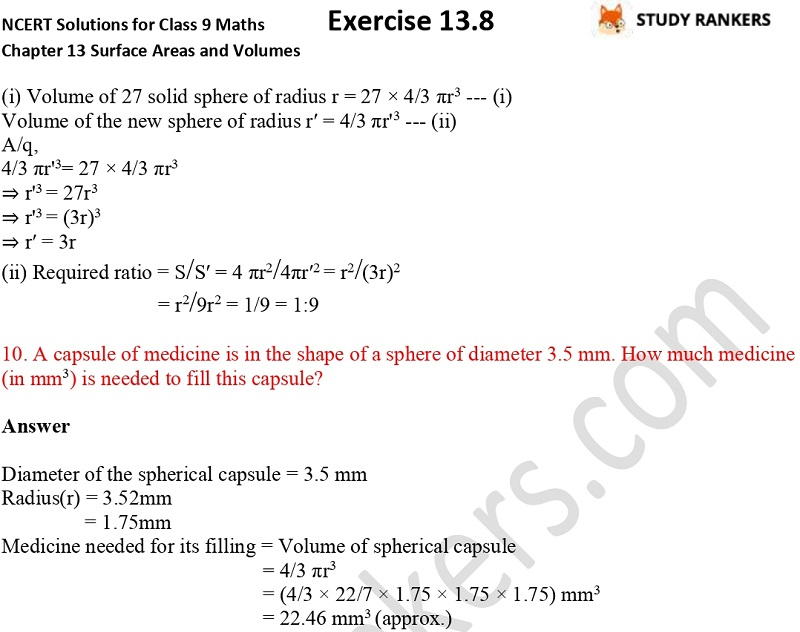>

## NCERT Solutions for Class 9 Maths Chapter 13 Surface Areas and Volumes Exercise 13.8

If you want accurate Chapter 13 Surface Areas and Volumes Exercise 13.8 Class 9 Maths NCERT Solutions then you can get them here. NCERT Solutions for Class 9 Maths prepared by Studyrankers experts that are detailed so any student can understand the basic concepts embedded in a question. These will be very much helpful in completing your homework and boosting marks in the examinations. These are updated as per the latest syllabus of CBSE.

Exercise 13.8 has total of 10 questions which are about finding the volume of a sphere, the amount of water displaced by a solid spherical ball etc.X